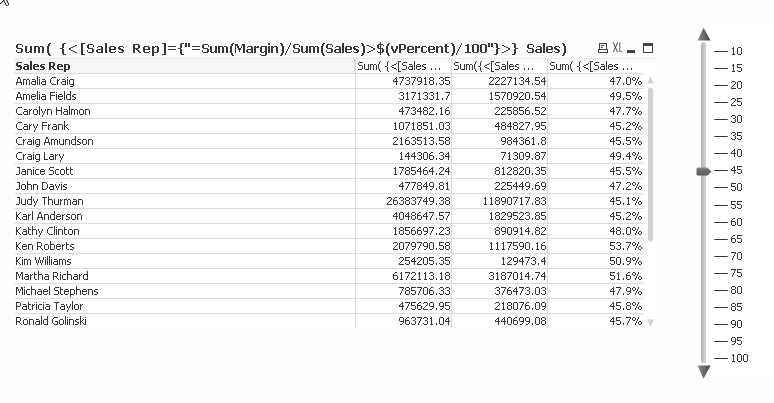QlikView App Dev

Discussion Board for collaboration related to QlikView App Development.

Announcements
QlikWorld 2022, LIVE in Denver CO., May 16-19, 2022. REGISTER NOW TO RECEIVE EARLY BIRD PRICING
cancel
Showing results for
Did you mean:Partner

Hide rows in pivot table.

Hi All,

I have a pivot table with an alternate state.The user can select 2 different periods to compare.

I'va also created a slider.

Is there a way that I can only show the rows with a percentage higher or equal to the selected % in the slider?Regards,

Kris

1 Solution

Accepted SolutionsPartner

It would have to not include the outer curly braces:

vPctAbove = '<[Sales Rep]={"=Sum(Margin)/Sum(Sales)>\$(vPercent)/100"}>'

Then you could do:

Sum( {State1\$(vPctAbove)} Quantity )

or maybe even a more reusable way:

vPctAbove = '[Sales Rep]={"=Sum(Margin)/Sum(Sales)>\$(vPercent)/100"}'

and

Sum( { State1 <\$(vPctAbove)> } Quantity )

5 RepliesMVP

Could be possible from 2 different selection with 2 different objects. Can you post example

Before develop something, think If placed (The Right information | To the right people | At the Right time | In the Right place | With the Right context)Partner

Yes by using a set expression in all the expressions:This the set expression which is similar for all expressions could be stored in a variable too so it is simpler to maintain and use in multiple expressions:

vPctAbove = '{<[Sales Rep]={"=Sum(Margin)/Sum(Sales)>\$(vPercent)/100"}>}'

Then the expression for Sum(Sales) should be Sum( \$(vPctAbove) Sales)

for Sum(Margin)/Sum(Sales) it wold be Sum( \$(PctAbove) Margin) / Sum(Sales)

The hiding relies on the fact that all rows which contains zeros in all the expressions will be excluded.

I did the test and example in the "Data Visualization" example QlikView app that comes with QlikView to illustrate and prove my point since you didn't provide any test data.Partner
Author

Thanks Petter,

It works.

But how should I use the variable vPctAbove in my expression with the alternate state?

sum( {State1}  Quantity)Partner

It would have to not include the outer curly braces:

vPctAbove = '<[Sales Rep]={"=Sum(Margin)/Sum(Sales)>\$(vPercent)/100"}>'

Then you could do:

Sum( {State1\$(vPctAbove)} Quantity )

or maybe even a more reusable way:

vPctAbove = '[Sales Rep]={"=Sum(Margin)/Sum(Sales)>\$(vPercent)/100"}'

and

Sum( { State1 <\$(vPctAbove)> } Quantity )Partner
Author

Thanks Petter!!

It is working fine for me.Community Browser